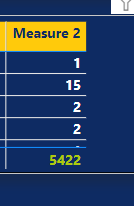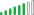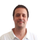cancel
Showing results for
Did you mean:

Fabric is Generally Available. Browse Fabric Presentations. Work towards your Fabric certification with the Cloud Skills Challenge.Helper II

## how to get a total of a value in a dax power bi

Hi,

I have a measure that calculates the count of a categoric value.I want to calculate the overall percentage of this measure.

For example;

1/5422

15/5422

2/5422 and so on..

Here is my DAX;

`Measure 2 = var reason = CALCULATE(COUNT('Table'[Reason]))returnreason`

I can't write the var function that will always be 5422. Its the total countrows of the ID column.

2 ACCEPTED SOLUTIONSSolution Supplier

You are wright, it cant be in a variable.

``````% of Count =
DIVIDE(
COUNTROWS(Table),
CALCULATE(COUNTROWS(Table),
ALL(table)
)
)``````

Kind regards,
JoséHelper II

I used ALLSELECTED()  an it worked. Thanks Jose big time.

10 REPLIES 10Solution Supplier

Hi

You need the folowing measure

``````% of Count =

var _count = COUNTROWS(Table[Reason])

RETURN
DIVIDE(
_count,
CALCULATE(_count,
ALL(table)
)
)``````

Kind regards,
JoséHelper II

Hi Jose,

Thank you for the response.

Unfortunately,

I have an error in this row of the DAX:

`var _count = COUNTROWS(Table[Reason])`

Because countrows only takes table as a function.Solution Supplier

My bad. Then count the table rows

``````% of Count =

var _count = COUNTROWS(Table)

RETURN
DIVIDE(
_count,
CALCULATE(_count,
ALL(table)
)
)``````

Kind regards,
JoséHelper II

Hi again,

Row based, it is always will be 1/1, 15/15, and so on . So, I get always %100. It doesnt get the total row count which is 5422. Total row count shouldnt change for every row.Solution Supplier

With that measure it should't. Have you tried it?

ALL() function  should remove all the filter contex and return the count of all rows.Helper II

Yes, I tried it. I am adding this measure to a table. Could that be the issue? Because the main idea is the give perc of the specific row's category.Solution Supplier

You are wright, it cant be in a variable.

``````% of Count =
DIVIDE(
COUNTROWS(Table),
CALCULATE(COUNTROWS(Table),
ALL(table)
)
)``````

Kind regards,
JoséResponsive Resident

Nice one, Zé! 🙂Helper II

It seems working but need a little bit more. Can we get the total rows with the selected filters on the table?

Lets say without filters we have 120k, but with filters we have 5422. Should I use KEEPFILTERS?Helper II

I used ALLSELECTED()  an it worked. Thanks Jose big time.Announcements#### Power BI Monthly Update - November 2023

Check out the November 2023 Power BI update to learn about new features.#### The largest Power BI and Fabric virtual conference

130+ sessions, 130+ speakers, Product managers, MVPs, and experts. All about Power BI and Fabric. Attend online or watch the recordings.Top Solution Authors
Top Kudoed Authors
Users online (6,363)